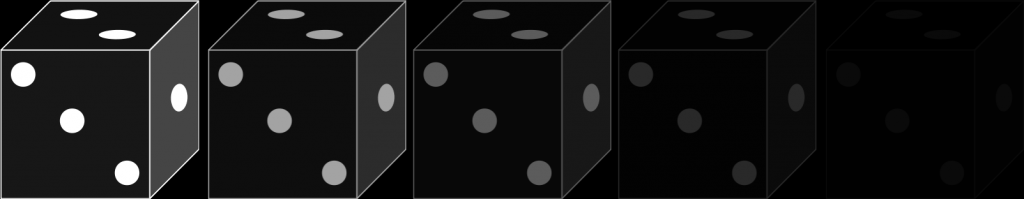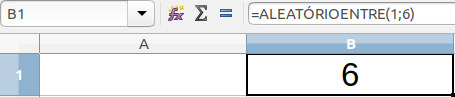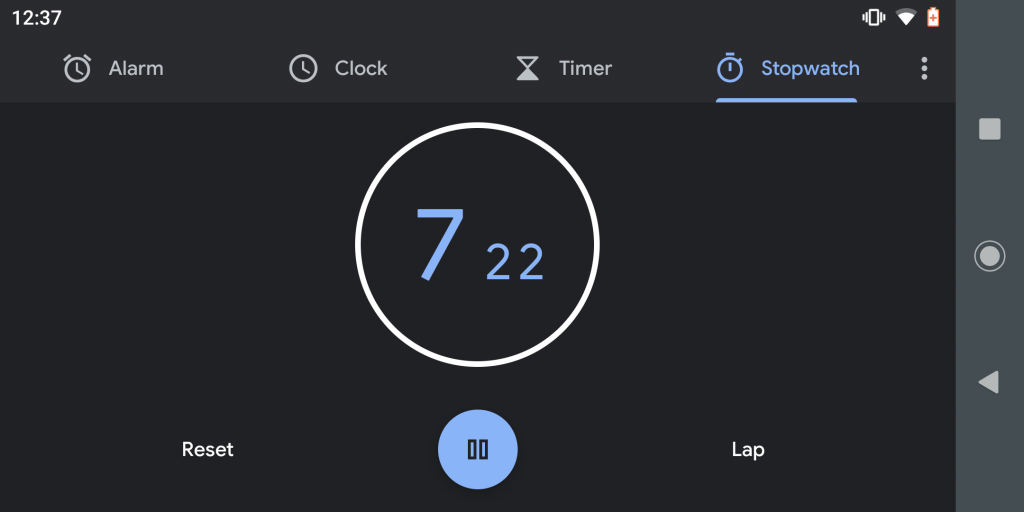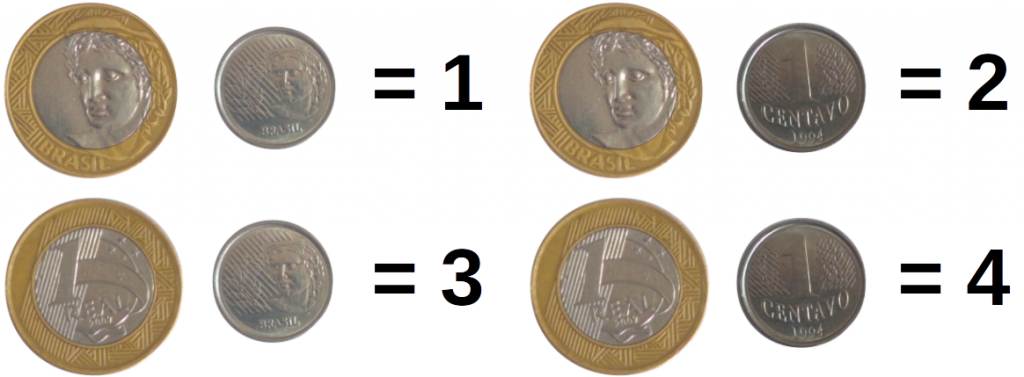# I lost my dices, can I still play?

Board / table games are great pastimes for periods of lethargy … but what about when we start playing, but we realize that the dice are gone? We may have kept it in another box, borrowed it, used it for other purposes, or even entered that list of things that disappear mysteriously without any explanation. We are not in the mood to leave the house looking for new dice and sometimes we can’t even do that. So what do we have to do in the face of this crisis?There are several dice simulators available on the internet, in fact they are basically customized random number generators. There we can generate most of the dice we need for our games, some of which are even available as mobile applications. Another solution is to create a dice simulator from a simple computer program like a spreadsheet. Simulating a dice is nothing more than creating a cell in the spreadsheet capable of generating a random number between 1 and the total faces of your original dice. A simple solution to the problem of missing dice to play.Example of 6-sided dice in LibreOffice Calc.

But let’s get to a more interesting situation. In the absence of sophisticated electronic resources, that is, we are without electricity and internet, but we really want to play, can you simulate the dice accurately using other methods?

To simulate dice is to identify a probability event with equal possibilities and apparently fair. For example, RPG players often use the set of two 10-sided dice, one of which represents the tens and the other the unit. With this they determine values between 1 and 100. We can simulate this same effect from a common watch / cell phone timer that marks at least the hundredths. I say that this is an apparently fair system, because if we start the timer, wait at least about 5 seconds without looking at its display and then pause it, we will hardly know how many hundredths will be dialing at that moment. With this method we have for hundredths in 00 the equivalent of results in 100 for the two 10-sided dice.Another way to simulate dice is, for example, with 4-sided dice. They are again quite common in role-playing games. We can simulate your result from two common currencies. Just consider your order when playing, or even assign different currencies for the occasion.

For example, I used a 1 dollar coin and a 1 cent coin. The 1 dollar coin is worth 0 and the crown is worth 2, while the 1 cent coin is worth 0 and the crown is 1. With this we have the sum of the values generating 4 equally probable results {0, 1, 2, 3}. To adjust to the traditional 4-sided dice, we add 1 to the final result, and thus we can obtain their respective values {1, 2, 3, 4}.Now let’s discuss how to simulate 6-sided dice. In an isolated case, we can write numbers from 1 to 6 on six papers, crumple them in a glass and take one of them at random. This will generate an event with an equal probability of obtaining numbers between 1 and 6. We can, for example, reproduce this object the number of times that corresponds to the necessary dice, reaching 2, 3, 4 … cups to equal the same amount of dice. However, there are more “fun” ways to do this without using so many glasses.

The problem with rolling two 6-sided dice, for example, is increasing the number of options, because instead of 6, we now have 36 options. To simulate this event we could take the cards from a conventional deck in a rudimentary way, that is, from 1 to 10 of 3 suits (thus equivalent from 1 to 30) and from 1 to 6 of a fourth suit (thus equivalent from 31 to 36 ). Shuffling it and drawing one of the 36 cards, we come to an event equally likely to roll 2 dice.

With fewer cards, we could reduce our deck to two, each containing 6 cards and serve the same way as cups. Thus, each deck of 6 cards would be equivalent to a 6-sided die. This, despite working, is also not as exciting as rolling 3 dice at the same time … imagining a War game, in which two players, each with at least 4 armies in their territory face each other, this results in a 3 dice roll 6 faces per player. Removing them from the hills may not be as interesting as the suggestion below.

We can improve things a bit if we have a complete conventional deck of 54 cards (13 of each suit and 2 wildcards, one red and one black). Dividing it in two we have 27 cards for each player. 27 cards sorted into their own sets, added to 3 coins for each player (considering their order as mentioned above), we will have 8 possibilities for the coins and 27 for the cards, therefore, 216 possibilities. This is analogous to the total possibilities of throwing 3 6-sided dice.

It only remains to build a bijector relation for each result of the dice of both players with each result of the cards + coins, for example:

Now let’s discuss how to simulate 6-sided dice. In an isolated case, we can write numbers from 1 to 6 on six papers, crumple them in a glass and take one of them at random. This will generate an event with an equal probability of obtaining numbers between 1 and 6. We can, for example, reproduce this object the number of times that corresponds to the necessary dice, reaching 2, 3, 4 … cups to equal the same amount of dice. However, there are more “fun” ways to do this without using so many glasses.

The problem with rolling two 6-sided dice, for example, is increasing the number of options, because instead of 6, we now have 36 options. To simulate this event we could take the cards from a conventional deck in a rudimentary way, that is, from 1 to 10 of 3 suits (thus equivalent from 1 to 30) and from 1 to 6 of a fourth suit (thus equivalent from 31 to 36 ). Shuffling it and drawing one of the 36 cards, we come to an event equally likely to roll 2 dice.

With fewer cards, we could reduce our deck to two, each containing 6 cards and serve the same way as cups. Thus, each deck of 6 cards would be equivalent to a 6-sided die. This, despite working, is also not as exciting as rolling 3 dice at the same time … imagining a War game, in which two players, each with at least 4 armies in their territory face each other, this results in a 3 dice roll 6 faces per player. Removing them from the hills may not be as interesting as the suggestion below.

We can improve things a bit if we have a complete conventional deck of 54 cards (13 of each suit and 2 wildcards, one red and one black). Dividing it in two we have 27 cards for each player. 27 cards sorted into their own sets, added to 3 coins for each player (considering their order as mentioned above), we will have 8 possibilities for the coins and 27 for the cards, therefore, 216 possibilities. This is analogous to the total possibilities of throwing 3 6-sided dice.

It only remains to build a bijector relation for each result of the dice of both players with each result of the cards + coins, for example: